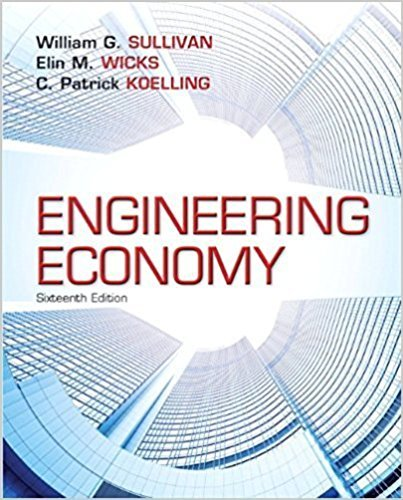×
Log in to StudySoup
Get Full Access to Engineering Economy (1) - 16 Edition - Chapter 18 - Problem 18.2
Join StudySoup for FREE
Get Full Access to Engineering Economy (1) - 16 Edition - Chapter 18 - Problem 18.2

Already have an account? Login here
×
Reset your password

# A company that manufactures high-speedISBN: 9780133439274 207

## Solution for problem 18.2 Chapter 18

Engineering Economy (1) | 16th Edition

• Textbook Solutions
• 2901 Step-by-step solutions solved by professors and subject experts
• Get 24/7 help from StudySoup virtual teaching assistantsEngineering Economy (1) | 16th Edition

4 5 1 294 Reviews
31
4
Problem 18.2

A company that manufactures high-speed submersiblerotary-indexing spindles is consideringan upgrade of production equipment to reducecosts over the next 5 years. The company can invest\$80,000 now, 1 year from now, or 2 yearsfrom now. Depending on when the investment ismade, the savings will vary. The saving estimatesare \$26,000, \$31,000, or \$37,000 per year if theinvestment is made now, 1 year from now, or 2years from now, respectively. The company willonly invest if the ROR is at least 20% per year. Using a future worth analysis, determine if thetiming of the investment will affect the return requirementand, if so, when the investment shouldbe made. Solve by (a) hand, and (b) spreadsheet.

Step-by-Step Solution:
Step 1 of 3

ENGR 121 B Lecture Notes for 10/31/2016 Spencer Kociba ● Calculating Standard Deviation ○ u=mean, N=number of elements in a vector N ○ SD= 1 * ∑(x(i) − u) √ N−1 k=1 ■ Take each element, subtract the mean and square it. Repeat this for all the elements in the vector and sum them. Divide by N-1 and take the square root ○ Function this_std=standard_deviation(vec) ○ Part 1 (calculating the mean) ■ sum=0 ■ For i=1:length(vec) ● sum=sum+vec(i) ■ End ■ this_mean=sum/length(vec) ○ Part 2 (calculation of SD) ■ For i=1:len

Step 2 of 3

Step 3 of 3

##### ISBN: 9780133439274

Unlock Textbook Solution

Enter your email below to unlock your verified solution to:

A company that manufactures high-speed